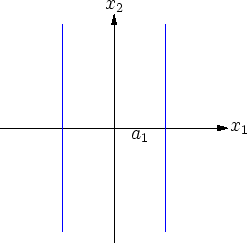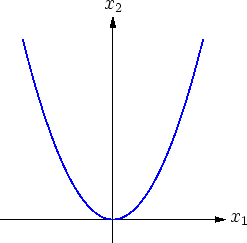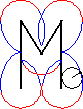[home] [lexicon] [problems] [tests] [courses] [auxiliaries] [notes] [staff]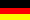Mathematics-Online lexicon:

# Euclidean Normal Forms of Two-Dimensional Quadrics

 A B C D E F G H I J K L M N O P Q R S T U V W X Y Z overview

There exist appropriate Cartesian coordinate systems with respect to which the equations defining quadrics have the following normal forms.

 normal form name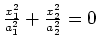point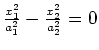intersecting pair of lines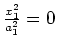coincident lines

 normal form name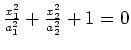(empty set)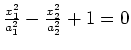hyperbola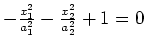ellipse(empty set)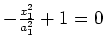parallel pair of lines

 normal form name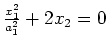parabola

The normal forms are uniquely determined up to permutation of subscripts and in the case of conical quadrics up to multiplication by a constant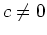.

The valuesare set to be positive and are called lengths of the principal axes of the quadric.

 intersecting pair of lines coincident lines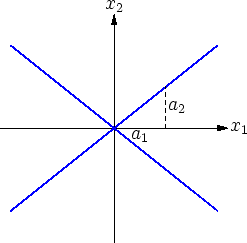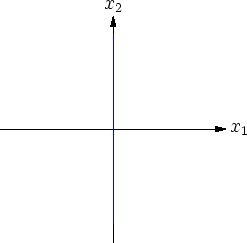hyperbola ellipse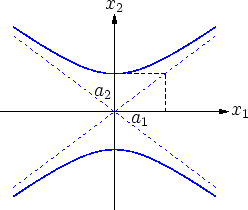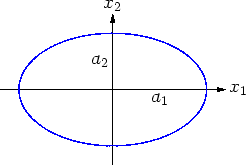parallel pair of lines parabola